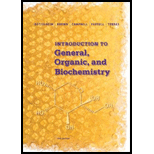# Problem 4-16 In an experiment to prepare aspirin, the theoretical yield is 153.7 g. if the actual yield is 124.3 g, what is the percent yield?### Introduction to General, Organic a...

11th Edition
Frederick A. Bettelheim + 4 others
Publisher: Cengage Learning
ISBN: 9781285869759### Introduction to General, Organic a...

11th Edition
Frederick A. Bettelheim + 4 others
Publisher: Cengage Learning
ISBN: 9781285869759

#### Solutions

Chapter
Section
Chapter 4.7, Problem 4.16P
Textbook Problem

## Problem 4-16 In an experiment to prepare aspirin, the theoretical yield is 153.7 g. if the actual yield is 124.3 g, what is the percent yield?

Expert Solution
Interpretation Introduction

Interpretation:

Percent yield of aspirin should be identified.

Concept Introduction:

Percent yield is the determination of total outcome of the reaction through which amount of product is produced from reactant. It can be determined as follows:

Percent yield = Actual yeild theoretical yield × 100.

### Explanation of Solution

Percent yield = Percent yield = Actual yeild theoretical yield × 100= Perc

### Want to see the full answer?

Check out a sample textbook solution.See solution

### Want to see this answer and more?

Bartleby provides explanations to thousands of textbook problems written by our experts, many with advanced degrees!

See solution